# SPSS tutorials

BASICS DATA ANALYSIS T-TEST ANOVA CHI-SQUARE TEST

# How to Draw a Regression Line in SPSS?

This tutorial shows how to draw a regression line in SPSS. We encourage you to follow along by downloading and opening job_performance.sav, part of which are shown below.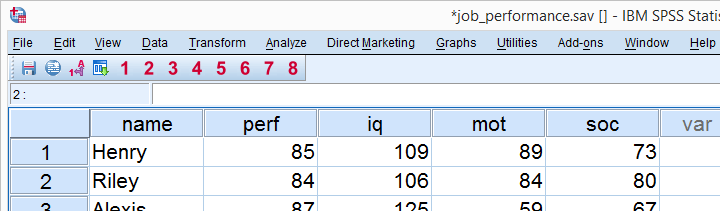Our data basically just hold job performance scores and IQ, motivation and social support which -supposedly- contribute to job performance. The meaning of our variables is seen under variable view, part of which is shown below.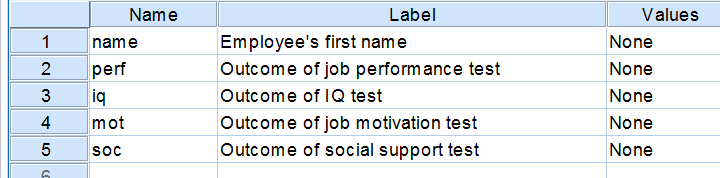For these data, we'd normally inspect all predictors simultaneously by means of multiple regression. This tutorial, however, will be limited to the relation between motivation and job performance. That is, we'll ignore IQ and social support for now.

## Regression Line Step 1: Scatterplot

So what does the relation between job performance and motivation look like? The best way to find out is running a scatterplot of these two variables as shown below. After doing so, we'll add a linear regression line to our plot to see whether it reasonably fits our data points.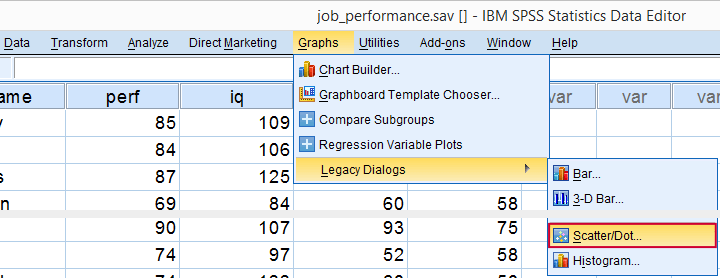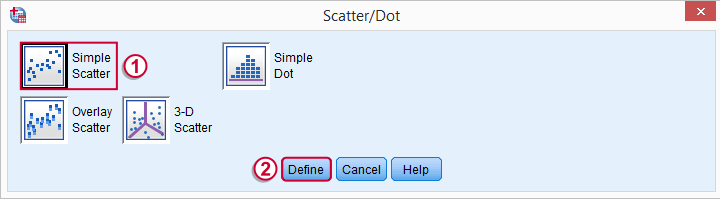As shown below, we usually plot the data values of our dependent variable on the y-axis.You could throw in a title at this point but we'll skip that for now.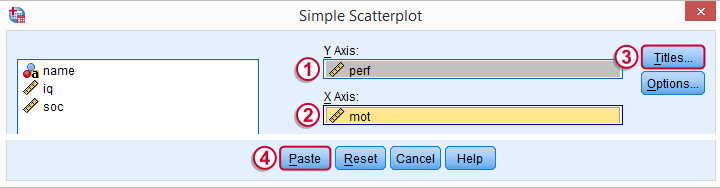## SPSS Basic Scatterplot Syntax

Completing the steps shown in the previous screenshots results in the syntax below.

*Simple scatterplot with performance on y-axis, motivation on x-axis.

GRAPH
/SCATTERPLOT(BIVAR)=mot WITH perf
/MISSING=LISTWISE.

## Step 2: Adding the Regression Line

Double-clicking our scatterplot in the output viewer window will open it in a Chart Editor window. Navigating to ElementsFit line at total immediately adds the desired regression line to our scatterplot. We don't have to change any of the default settings; we can just the dialog.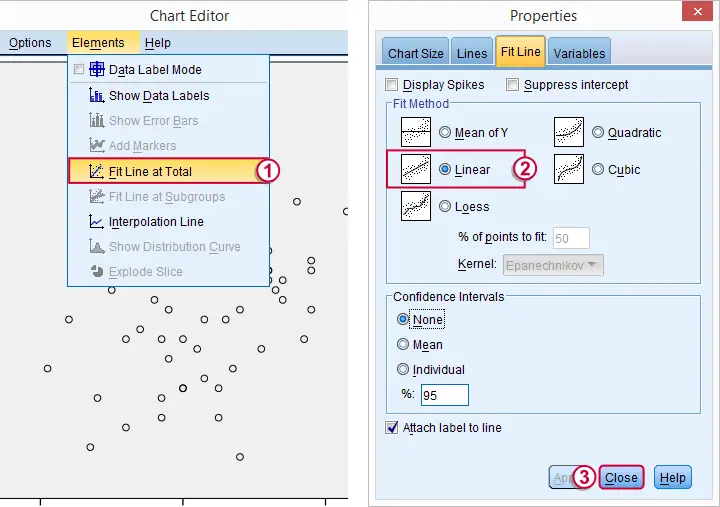## Result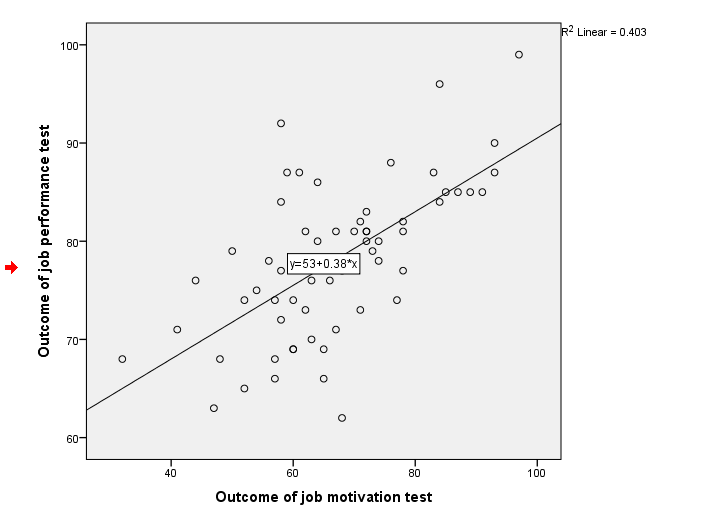For a prettier chart, you could manually style it somewhat -again, double-click it for opening the Chart Editor. We tried to create an SPSS chart template for styling our scatterplot with a regression line but the newly added elements weren't affected by it so it didn't work.

## But are the Results Correct?

The ease with which we added our regression line without actually running REGRESSION made us a bit suspicious about the results. The syntax below -generated from AnalyzeRegression- should yield a regression equation identical to the one in our scatterplot.

*Simple regression for double checking regression line results.

REGRESSION
/MISSING LISTWISE
/STATISTICS COEFF OUTS R ANOVA
/CRITERIA=PIN(.05) POUT(.10)
/NOORIGIN
/DEPENDENT perf
/METHOD=ENTER mot.

## Results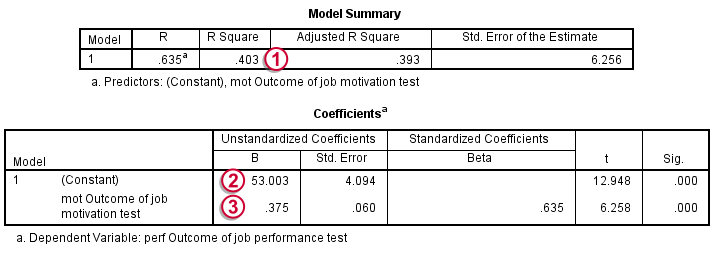We find ther square value in our scatterplot in the Model Summary table (keep in mind that we usually prefer R-square adjusted instead).,The unstandardized coefficients in our Coefficients table also correspond to our scatterplot. We can be confident about the regression line we added to it.

## Final Note

Now what if I need 10 regression lines? Or 100? I surely don't want to process 100 scatterplots manually. Unfortunately, I didn't find any way for adding them by syntax. If anyone has a suggestion on that, I'd love to hear it!

# Let me know what you think!

*Required field. Your comment will show up after approval from a moderator.

# This tutorial has 23 comments

•### By Edward Sternin on March 1st, 2019

Alternatively, calculate the line of best fit right in gpl:

ELEMENT: line( position( smooth.linear(l*w) ), color(color.red))

•### By Edward Sternin on March 1st, 2019

GGRAPH
/GRAPHDATASET NAME="gdata" VARIABLES=length weight
/GRAPHSPEC SOURCE=INLINE.
BEGIN GPL
SOURCE: s=userSource(id("gdata"))
DATA: l=col(source(s), name("length"))
DATA: w=col(source(s), name("weight"))
GUIDE: axis(dim(1), label("birth length, cm"))
GUIDE: axis(dim(2), label("birth weight, g"))
ELEMENT: point(position(l*w))
TRANS: y1 = eval((l-32)*65+800)
ELEMENT: line(position(l*y1), color(color.green))
TRANS: y2 = eval(l*51.239-760.524)
ELEMENT: line(position(l*y2), color(color.blue))
TRANS: y3 = eval(l*79.889-1864.270)
ELEMENT: line(position(l*y3), color(color.red))
END GPL.

•### By Edward Sternin on March 1st, 2019

* length weight
41.00 1360.00
40.00 1490.00
38.00 1490.00
38.00 1180.00
38.00 1200.00
32.00 680.00
33.00 620.00
38.00 1060.00
30.00 1320.00
34.00 830.00
32.00 880.00
39.00 1130.00
38.00 1140.00
39.00 1350.00
37.00 950.00
39.00 1220.00
38.00 980.00
42.00 1480.00
39.00 1250.00
38.00 1250.00

•### By Ruben Geert van den Berg on January 17th, 2019

Hi Erik!

That's interesting! What you refer to is a Python based extension that's included with the Python essentials. Indeed, I no longer see the dialogs in version 25 either. Strange indeed.

Also note that the chart builder -starting from version 25- can create syntax for a scatterplot with a regression line, possibly for subgroups. The latter option comes in handy if you're looking for interaction effects.

•### By Erik Mønness on January 17th, 2019

These commands generate 2 overlayed regression plots

STATS REGRESS PLOT YVARS=Lonn15 XVARS=Aar COLOR=Kjonn
/OPTIONS CATEGORICAL=BARS GROUP=1 INDENT=15 YSCALE=75
/FITLINES LINEAR APPLYTO=GROUP.

In version24, ther was a Regression plot In version 25, the menue is removed, but the commands work.